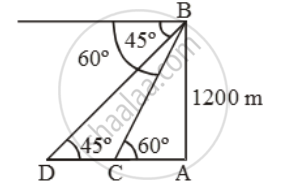# The Pilot of a Helicopter, at an Altitude of 1200m Finds that the Two Ships Are Sailing Towards It in the Same Direction. the Angle of Depression of the Ships as Observed from the Helicopter Are 60º - Mathematics

Sum

The pilot of a helicopter, at an altitude of 1200m finds that the two ships are sailing towards it in the same direction. The angle of depression of the ships as observed from the helicopter are 60º and 45º respectively. Find the distance between the two ships

#### Solution

Let B the position of the helicopter and let C, D be the ships.

Let AB be the vertical height.Then, AB = 1200 m,

∠ACB = 60º and ∠ADB = 45º.

\text{Then, }text{ }\frac{AD}{AB}=\text{cot 45}^\text{o}text{ = 1}

\Rightarrow \frac{AD}{1200}=1\Rightarrow \text{AD = 1200 m}

\text{And, }text{ }\frac{AC}{AB}=\text{cot 60}^\text{o}=\frac{1}{\sqrt{3}}\text{ }

\Rightarrow \frac{AC}{1200}=\frac{1}{\sqrt{3}}\text{ }

\Rightarrow AC=\frac{1200}{\sqrt{3}}=400\sqrt{3}\text{ m}\text{.}

Concept: Heights and Distances
Is there an error in this question or solution?